October 20, 2020

# Main Collections Traits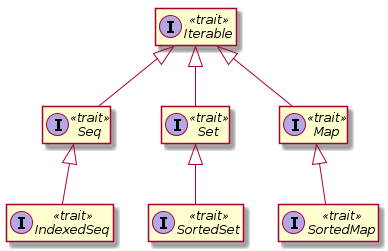# `Iterable`s

An `Iterable` is any collection that can yield an `Iterator`, which allows you to systematically access each element of the collection (see the Iterator Pattern).

### Example

``````val coll = Array(1, 7, 2, 9) // some Iterable
val iter = coll.iterator
while (iter.hasNext)
println(iter.next())``````

# `Seq`uences

• A `Seq` is an ordered sequence of values like an array or a `List`.
• An `IndexedSeq` allows fast random access through an integer index.

### Example

An `ArrayBuffer` is an `IndexedSeq` but a `LinkedList` is not.

# `Set`s

• A `Set` is an unordered collection of values.
• A `SortedSet` maintains an sorted visitation order.
• Elements are traversed in sorted order.

# `Map`s

• A `Map` is a set of (key, value) pairs.
• A `SortedMap` maintains a sorted visitation order by the keys.

# Constructing Collections

Each Scala collection trait or class has a companion object with an `apply` method that constructs instances of the collection.

### Example

``````Iterable(0xFF, 0xFF00, 0xFF0000)
Set(Color.RED, Color.GREEN, Color.BLUE)
Map(Color.RED -> 0xFF0000, Color.GREEN -> 0xFF00, Color.BLUE -> 0xFF)
SortedSet("Hello", "World")``````

# Converting Between Collection Types

• Can use `toSeq`, `toSet`, `toMap`, etc. to convert between collection types.
• There is also a generic `to[C]` for type `C` (the target collection type).
``````val col = collection.mutable.ArrayBuffer(1, 1, 2, -4, 2, 100)
val set = col.toSet
val list = col.to[List]``````

# Mutable and Immutable Collections

• As we have seen previously, you can make both mutable and immutable collection objects.
• An immutable collection never changes.
• Allows safe reference sharing in concurrent programs.
• No data races since there are no (concurrent) data writes.

### Example

• `scala.collection.mutable.Map`
• `scala.collection.immutable.Map`

### Immutability Preference

The preference is to immutability.

#### Example

``````val map = Map("Hello" -> 42) // : Map[String, Int] = Map("Hello" -> 42)
map.getClass() // : Class[T] = class scala.collection.immutable.Map\$Map1``````

# Using Immutable Collections

Compute the set of all digits of an integer:

``````def digits(n: Int): Set[Int] = n match {
case _ if n < 0 => digits(-n)
case _ if n < 10 => Set(n)
case _ => digits(n / 10) + (n % 10)
}

digits(1729) // : Set[Int] = Set(1, 7, 2, 9)``````

# Sequences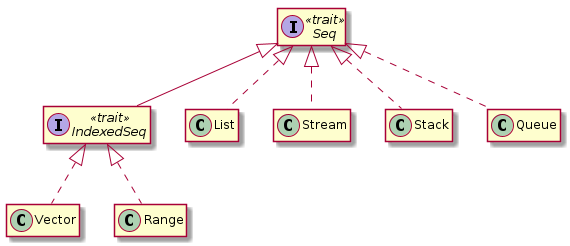• A `Vector` is the immutable equivalent of an `ArrayBuffer`.
• A `Range` is an integer sequence, e.g., `1 to 10` or `10.to(30, 10)`.

# Lists

• A list is either `Nil` (empty) or an object with a `head` and a `tail`.
• The `tail` is also a `List`.
• Similar to the `car` and `cdr` operations in Lisp.
``````val lst = List(4, 2) // : List[Int] = List(4, 2)
lst.head // : Int = 4
lst.tail // : List[Int] = List(2)
lst.tail.head // : Int = 2
lst.tail.tail // : List[Int] = List()``````

### Creating `List`s

You can use `::` to create a `List` with a given `head` and `tail`:

``````9 :: List(4, 2)
9 :: 4 :: 2 :: Nil
9 :: (4 :: (2 :: Nil)) // right-associative.``````
• All of these create a `List(9, 4, 2)`.
• Similar to the `cons` operator in Lisp for constructing lists.

# Summing `List`s

Natural fit for recursion:

``````def sum(lst: List[Int]): Int =
if (lst == Nil) 0 else lst.head + sum(lst.tail)

sum(List(9, 4, 2)) // : Int = 15``````

Use pattern matching:

``````def sum(lst: List[Int]): Int = lst match {
case Nil => 0
case h :: t => h + sum(t) // h is lst.head, t is lst.tail
}

sum(List(9, 4, 2)) // : Int = 15``````

This is just for demonstration purposes. Should really just use the built-in method:

``List(9, 4, 2).sum // : Int = 15``

# `Set`s

• A `Set` is an unordered collection of unique elements.
• Adding an element to a `Set` that already exists in the `Set` has no effect.
``````Set(2, 0, 1) + 1 == Set(2, 0, 1) // : Boolean = true
Set(2, 0, 1) + 4 == Set(2, 0, 1, 4) // : Boolean = true``````
• Ordering is not guaranteed.
• By default, implemented as hash sets.
• Constant-time look-up.

### `LinkedHashSet`s

Elements are traversed in the order for which they were inserted:

``````val weekdays = scala.collection.mutable.LinkedHashSet("Mo", "Tu", "We", "Th", "Fr")
weekdays.mkString(", ") // : String = Mo, Tu, We, Th, F``````

### `SortedSet`s

Elements are traversed in the sorted order:

``````val nums = collection.immutable.SortedSet(5, 2, -2, 5, 2, -100)
nums.mkString(", ") // : String = -100, -2, 2, 5``````

# `Set` Operations

### Containment

``````val digits = Set(1, 7, 2, 9)
digits contains 0 // : Boolean = false``````

### Subset

``Set(1, 2) subsetOf digits // : Boolean = true``

### Union, Intersection, and Set Difference

``````val primes = Set(2, 3, 5, 7)
digits union primes // : Set[Int] = Set(5, 1, 9, 2, 7, 3)
digits & primes // : Set[Int] = Set(7, 2)
digits -- primes // : Set[Int] = Set(1, 9)``````

# Operations for Adding or Removing Elements

#### Immutable Collections

• `+` is used for adding elements to unordered collections.
``````var numberSet = Set(1, 2, 3)
numberSet += 5 // Sets numberSet to the immutable set numberSet + 5``````
• `+:` and `:+` are for pre-pending and appending elements to ordered collections, respectively.
``````Vector(1, 2, 3) :+ 5  // : Vector[Int] = Vector(1, 2, 3, 5)
1 +: Vector(1, 2, 3) // : Vector[Int] = Vector(1, 1, 2, 3)``````

#### Mutable Collections

``````val numbers = ArrayBuffer(1, 2, 3) // : ArrayBuffer[Int] = ArrayBuffer(1, 2, 3)
numbers += 5 // : ArrayBuffer[Int] = ArrayBuffer(1, 2, 3, 5)``````

### Removing Elements

``Set(1, 2, 3) - 2 // : Set[Int] = Set(1, 3)``

### Working With Multiple Elements

``````numbers ++ Vector(1, 2, 7, 9) // : Vector[Int] = Vector(1, 2, 3, 5, 1, 2, 7, 9)
numbers -- Vector(1, 2, 7, 9) // : Vector[Int] = Vector(3, 5)``````

# Mapping a Function

• Used to transform all elements of a collection.
• `map` applies a function to each element of a collection and results in another collection containing the mapped elements.
``````val names = List("Peter", "Paul", "Mary")
names.map(_.toUpperCase) // List("PETER", "PAUL", "MARY")``````

Same as:

``for (n <- names) yield n.toUpperCase``

However, the `map` version is preferred as it is more easily parallelizable (more on this later.)

# Multi-level Mapping

If a function returns a collection, you can “flatten” each of these into a single result using `flatMap`.

Consider the following function that returns a collection:

``def ulcase(s: String) = Vector(s.toUpperCase(), s.toLowerCase())``

Now, let’s `map` each of the names to the value produced by this function:

``names.map(ulcase) // : List[Vector[String]] = Vector("PETER", "peter"), Vector("PAUL", "paul"), Vector("MARY", "mary")``

Suppose we just want a `List` of names rather than a `List` of `Vector`s of names:

``names.flatMap(ulcase) // : List[String] = List("PETER", "peter", "PAUL", "paul", "MARY", "mary")``

# Other Mapping

### Mutable Mapping

• The previous examples dealt with immutable collections.
• For mutable collections, use `transform` instead of `map`.

### Mapping Partial Functions

• `collect` works with partial functions, i.e., those that may not be defined for all inputs.
``"-3+4".collect { case '+' => 1 ; case '-' => -1 } // : Vector[Int] = Vector(-1, 1)``

### Applying Side-effecting Functions

To apply a function where the return value is not important (e.g., it returns `Unit`), use `foreach`:

``names.foreach(println)``

Outputs:

``````Peter
Paul
Mary``````

# Reducing

• The functions seen so far are unary, i.e., they operate on a single operand.
• We applied such functions to every element of the collection.
• What if we want to combine multiple values in some way, e.g., using a binary function, i.e., a function taking two operands?
• The call `c.reduceLeft(op)` applies `op` to successive elements.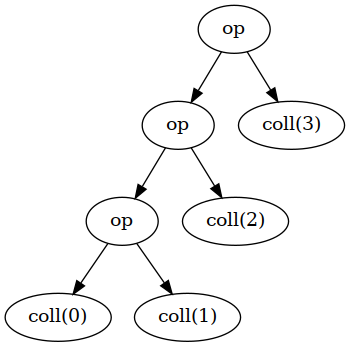# `reduceLeft`

``List(1, 7, 2, 9).reduceLeft(_ - _)``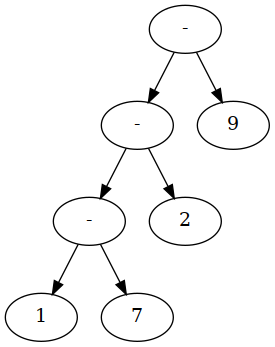Expands to: `((1 - 7) - 2) - 9 = 1 - 7 - 2 - 9 = -17`

# `reduceRight`

Does the same but starts at the end of the collection:

``List(1, 7, 2, 9).reduceRight(_ - _)``

Expands to: `1 - (7 - (2 - 9)) = 1 - 7 + 2 - 9 = -13`

# Folding

• Can start the computation with an initial element that is not part of the collection.
• Use `coll.foldLeft(init)(op)`.

QUESTION: What kind of method is this?

QUESTION: Why use this kind of method?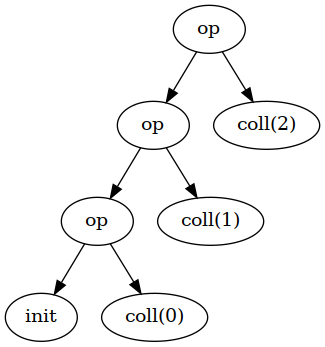### Example

``List(1, 7, 2, 9).foldLeft(0)(_ - _)``

Expands to: `0 - 1 - 7 - 2 - 9 = -19`

Can also be written as:

``(0 /: List(1, 7, 2, 9))(_ - _)``

QUESTION: What does the `/:` look like?

Count the frequencies of letters in a `String`.

• Visit each letter and update a mutable `Map`:
``````val freq = scala.collection.mutable.Map[Char, Int]()
for (c <- "Mississippi") freq(c) = freq.getOrElse(c, 0) + 1``````

After this code, `freq` becomes:

``collection.mutable.Map[Char,Int] = Map(M -> 1, s -> 4, p -> 2, i -> 4)``
• At each step, combine the frequency map and the newly encountered letter, producing a new frequency map:• LHS of `op` is the partially filled map.
• RHS of `op` is the new letter.
``````(Map[Char, Int]() /: "Mississippi") {
(m, c) => m + (c -> (m.getOrElse(c, 0) + 1))
}``````
• `Map[Char, Int]()` is the initial, empty map.
• Code in the block is the operation.
• The operation dictates how a new map is formed given a previous map and a new character.

# Scanning

• `scanLeft` and `scanRight` combine folding and mapping.
• Obtain a collection of all intermediate results:
``(1 to 10).scanLeft(0)(_ + _) // : collection.immutable.IndexedSeq[Int] = Vector(0, 1, 3, 6, 10, 15, 21, 28, 36, 45, 55)``

# Zipping

• In the preceding slides, we applied an operation to adjacent elements of a particular collection.
• Zipping is a way to deal with elements of multiple collections.

Suppose we want to combine the following two collections:

``````val prices = List(5.0, 20.0, 9.95)
val quantities = List(10, 2, 1)``````

Specifically, we want a list of pairs of corresponding elements from each list: `(5.0, 10), (20.0, 2), ...`.

``prices zip quantities // : List[(Double, Int)] = List((5.0,10), (20.0,2), (9.95,1))``

Apply a function to each pair:

``(prices zip quantities) map { p => p._1 * p._2 } // : List(50.0, 40.0, 9.95)``

Total price of all items:

``((prices zip quantities) map { p => p._1 * p._2 }) sum // : Double = 99.95``

# Zipping With Shorter Collections

• If one collection is shorter than the other, the resulting list has a number of elements equal to the shorter list:
``List(5.0, 20.0, 9.95) zip List(10, 2) /// : List[(Double, Int)] = List((5.0,10), (20.0,2))``
• Can specify defaults for the shorter list using `zipAll`:
• The two elements specify the defaults for whether the shorter list is the LHS or RHS.
``List(5.0, 20.0, 9.95).zipAll(List(10, 2), 0.0, 1) // : List[(Double, Int)] = List((5.0,10), (20.0,2), (9.95,1))``
• To correlate the collection indices with the elements, use `zipWithIndex`:
``"Scala".zipWithIndex // : collection.immutable.IndexedSeq[(Char, Int)] = Vector((S,0), (c,1), (a,2), (l,3), (a,4))``
• The “largest” pair:
``"Scala".zipWithIndex.max // : (Char, Int) = (l,3)``
• The index of the largest element:
``"Scala".zipWithIndex.max._2 // : Int = 3``

# Iterators

• Obtain an iterator from a collection with the `iterator` method.
• Not as commonly used as in C++ or Java.
• Iterators are useful for collections that are expensive to fully construct.

### Example

`Source.fromFile` returns an `iterator` to avoid reading an entire file into memory.

# Iterator Examples

### Obtaining an `Iterator`

``val iter = (1 to 10).sliding(3)``

### Using an `Iterator`

``````while (iter.hasNext)
println(iter.next())``````

Or:

``````for (elem <- iter)
println(elem)``````

#### Output

``````Vector(1, 2, 3)
Vector(2, 3, 4)
Vector(3, 4, 5)
Vector(4, 5, 6)
Vector(5, 6, 7)
Vector(6, 7, 8)
Vector(7, 8, 9)
Vector(8, 9, 10)``````

# Iterator Examples

``val iter = (1 to 10).sliding(3)``
``println(iter.length) // outputs 8.``

Obtaining the number of elements “exhausts” the iterator:

``println(iter.hasNext) // Returns false as the iterator is now consumed.``

### Convert an `Iterator` to a `Collection`

``````iter.toArray
iter.toIterable``````
• The collection starts at the current element.

# Peeking at the Next Element Returned By an `Iterator`

• You can look at the next element of an `Iterator` without consuming it.
``````val filename = "/usr/share/dict/words"
val iter = scala.io.Source.fromFile(filename).buffered

iter.next

# `LazyList`s

• Formerly called `Stream`s in Scala version 2.12.x.
• `Iterator`s are “lazy” alternatives to `Collection`s.
• Allows piecemeal traversal of `Collection`s, useful for large or computationally intensive collections to construct.
• Only compute the elements that are needed.
• `Iterator`s are fragile.
• Calls to `next` (and even `length`) change the iterator.
• `LazyList` implements an immutable linked list.
• It’s called “lazy” because it computes its elements only when they are needed.
• Because `LazyList`s compute their elements on-demand, they can be infinite collections!
• NOTE: For infinite sequences, some methods (such as `count`, `sum`, `max` or `min`) will not terminate.

# `LazyList` Examples

``def numsFrom(n: BigInt): LazyList[BigInt] = n #:: numsFrom(n + 1)``
• The `#::` operator is similar to `::` for `List`s.
``val tenOrMore = numsFrom(10) // : LazyList[BigInt] = LazyList(<not computed>)``
• As you see, no elements are initially computed.
• To compute the first element, call:
``tenOrMore.head // : BigInt = 10``
• The `tail` is unevaluated:
``tenOrMore.tail // : scala.collection.immutable.LazyList[BigInt] = LazyList(<not computed>)``
• To keep going, you need to get the `head` to compute values:
``tenOrMore.tail.tail.tail.head // : BigInt = 13``

# Operating on `LazyList`s

• Operations on `LazyList`s are deferred:
``````val squares = numsFrom(1).map(x => x * x)
// : scala.collection.immutable.LazyList[scala.math.BigInt] = LazyList(<not computed>)``````
• You must call `head` to force the evaluation of the next element:
``squares.head // : scala.math.BigInt = 1``
• To get multiple elements, you need to invoke operations that force the “realization” of the list.

• As the list may be expensive (or even impossible) to compute fully, you may not want to do this to the entire list:
``squares.take(5).foreach(println)``

Outputs:

``````1
4
9
16
25``````

Alternatively, `squares.take(5).force` results in `LazyList(1, 4, 9, 16, 25)`.

# Constructing `LazyList`s from `Iterator`s

• You can create a `LazyList` from an `Iterator`.

### Example

• `Source.getLines` returns `Iterator[String]`, which allows you to only visit each line once.
• In `LazyList`s, on the other hand, elements are memoized; that is, the value of each element is computed at most once.
• This allows you to revisit elements without incurring additional computation costs.
``````import scala.io.Source

val words = Source.fromFile("/usr/share/dict/words").getLines().to(LazyList)

words // : scala.collection.immutable.LazyList[String] = LazyList(<not computed>)
words.head // : String = A
words(5) // : String = AOL's
words // : scala.collection.immutable.LazyList[String] = LazyList(A, A's, AMD, AMD's, AOL, AOL's, <not computed>)``````
• Be cautious of memoization; it can eat up memory if you’re not careful.

# Lazy Views

• Besides `List`s, other kinds of `Collection`s can also be made “lazy” using the `view` method.
• The `view` method returns a `Collection` whose methods’ execution is deferred.

### Example

``````import scala.math._

val palindromicSquares = (1 to 1000000).view
.map(x => x * x)
.filter(x => x.toString == x.toString.reverse) // : scala.collection.View[Int] = View(<not computed>)``````
• Like `LazyList`s, this `View` also is not yet computed.
• The following call generates enough squares until ten palindromes have been discovered:
``palindromicSquares.take(10).mkString(",") // : String = 1,4,9,121,484,676,10201,12321,14641,40804``
• Unlike `LazyList`s, the results are not memoized.
• The computation starts over.
• Like `LazyList`s, the `force` method will force the computation of elements.
• Caution: The `apply` method forces evaluation of the entire collection.

# Mutating Lazy Views

• You may obtain a view into a part (slice) of a `Collection`.
• Any changes to that view reflect in the original `Collection`.

### Example

The following code will set the elements in the given slice to `0` but leave the remaining elements unchanged:

``````ArrayBuffer buffer = // ...
buffer.view(10, 20).transform(x => 0)``````

# Parallel Collections

• Writing correct concurrent programs is essential today yet difficult.
• May want to process large collections in parallel, using multiple cores at once.
• Some (e.g., commutative) operations parallelize naturally.

### Example

• To compute the sum of all elements, multiple threads can concurrently compute the sums of different parts of the collection.
• The partial results can be combined to obtain the sum of the entire collection.
• This is called divide-and-conquer (-and combine).

• Scheduling concurrent operations, like the one described above, can be burdensome and error-prone.
• Threads must be created (forked), spun (executed), and joined (have their results combined) in a synchronized fashion.
• Parallel collections makes this easier.

#### Example

To sum a large collection `coll` in parallel, simply write `coll.par.sum`.

# The `par` Method

• The `par` method of `Collection` results a parallel implementation of the `Collection.`
• If possible, the `Collection` implementation will execute parallel versions of its operations.

### Example

Count the even numbers in `coll` by evaluating the predicate on sub-collections in parallel and combine the results:

``coll.par.count(_ % 2 == 0)``

You can even parallelize `for` loops using `.par`:

``(0 until 100).par.foreach(i => print(s"\$i "))``

This prints the numbers in the order that the threads working on the task process them:

``25 26 27 28 29 30 37 12 13 14 15 16 17 43 44 45 46 47 48 49 41 42 6 7 8 9 10 11 3 4 5 1 2 75 76 77 78 79 80 81 82 83 84 85 86 87 88 89 90 91 92 93 94 95 96 97 98 99 62 63 64 65 66 67 68 69 70 71 72 73 74 56 57 58 59 60 61 53 54 55 51 52 50 0 40 18 19 20 21 38 39 31 22 23 24 32 33 34 35 36``

Compare this to the output of the sequential version, `(0 until 100).foreach(i => print(s"\$i "))`:

``0 1 2 3 4 5 6 7 8 9 10 11 12 13 14 15 16 17 18 19 20 21 22 23 24 25 26 27 28 29 30 31 32 33 34 35 36 37 38 39 40 41 42 43 44 45 46 47 48 49 50 51 52 53 54 55 56 57 58 59 60 61 62 63 64 65 66 67 68 69 70 71 72 73 74 75 76 77 78 79 80 81 82 83 84 85 86 87 88 89 90 91 92 93 94 95 96 97 98 99``

# Parallel Collections That Process In Order

• As previously stated, it is not always possible to apply the operations in parallel.
• A prominent example is when ordering of the collection elements must be maintained by the operation.
• In such cases, the parallel implementation cannot break the tasks up for efficient parallel execution.

### Example

``(0 until 100).par.mkString(" ") == (0 until 100).mkString(" ") // : Boolean = true``

# Parallel Collections and Side-Effects

You should never try to mutate shared (mutable) variables in parallel operations as the results are unpredictable.

``````// Don't do this:

var count = 0
coll.par.filter(_ % 2 == 0).foreach(i => count += 1)
count``````

### Example

In one run, `count` may be 186119. But, in another run of the same code, `count` may be 337736.

# Parallel Collections in Scala 2.13

• In Scala 2.13, parallel collections have moved into their own module.
• This was done so that programs not needing parallelism don’t have access to it.
• To work with parallel collections in Scala >= 2.13:
• Add a library dependency in `build.sbt`:
``````scalaVersion := "2.13.3"
libraryDependencies += "org.scala-lang.modules" %% "scala-parallel-collections" % "0.2.0"``````
``import scala.collection.parallel.CollectionConverters._``
• This will give you access to the `.par` methods, as well as the parallel collection hierarchy.

# Working With Parallel Collections

### Type Hierarchy

• You may think that parallel collections extend the sequential versions using inheritance.
• However, this is not the case.
• The objects returned from `.par` have types include the `ParSeq`, `ParSet`, and `ParMap` traits.
• They are not subtypes of `Seq`, `Set`, or `Map`.
• This means you cannot pass parallel collections to methods that expect sequential ones.

### Converting Between Parallel and Sequential

You can convert a parallel collection to a sequential one using `seq`:

``val result = coll.par.filter(p).seq``

# Associative Reduction and Folding

• As previously mentioned, not all operations are parallelizable, such as those maintaining data ordering.

• Examples include `reduceLeft` and `reduceRight`.

• Alternatively, you may use `reduce`, which operates on portions of the collection, combining the results.

• However, the given operation must be associative, i.e., fulfilling (a op b) op c = a op (b op c).

• Addition is associative but subtraction is not.
• Folding has a similar problem, and there is a `fold` method for that.

• Unfortunately, it is not as flexible as both arguments must be elements of the collection.

• For example, the following is allowed but more complex folding is not.
``val str = (1 to 1000).par.map(_.toString).fold("")(_ + _)``

Produces:

``val str: String = 12345678910111213141516171819202122232425262728...``

# Associative Aggregation

• You can use `aggregate` to solve the problem of complex parallel folding.
• It applies operations to portions of a collection and then uses another operation to combine the results.

### Example

``str.par.aggregate(Set[Char]())(_ + _, _ ++ _)``

is equivalent to:

``str.foldLeft(Set[Char]())(_ + _)``
• Both code snippets form a set of all distinct characters in `str`.
• Note that in Scala 2.13, `aggregate` is deprecated for sequential collections.

# Lab

1. Write a function that receives a collection of strings and a map from strings to integers. Return a collection of integers that are values of the map corresponding to one of the strings in the collection. For example, given `Array("Tom", "Fred", "Harry")` and `Map("Tom" -> 3, "Dick" -> 4, "Harry" -> 5)`, return `Array(3, 5)`. Hint: Use `flatMap` to combine the `Option` values returned by `get`.

2. The method `java.util.TimeZone.getAvailableIDs` yields time zones such as `Africa/Cairo` and `Asia/Chungking`. Which continent has the most time zones? Hint: `groupBy`.

3. Harry Hacker reads a file into a `String` and wants to use a parallel collection to update the letter frequencies concurrently on portions of the `String`. He uses the following code:

``````val frequencies = new scala.collection.mutable.HashMap[Char, Int]
for (c <- str.par) frequencies(c) = frequencies.getOrElse(c, 0) + 1``````

Why is this a terrible idea? How can he really parallelize the computation? (Hint: Use `aggregate`.)